# Support Vector Machine Algorithm

• Difficulty Level : Medium
• Last Updated : 22 Jan, 2021

Support Vector Machine(SVM) is a supervised machine learning algorithm used for both classification and regression. Though we say regression problems as well its best suited for classification. The objective of SVM algorithm is to find a hyperplane in an N-dimensional space that distinctly classifies the data points. The dimension of the hyperplane depends upon the number of features. If the number of input features is two, then the hyperplane is just a line. If the number of input features is three, then the hyperplane becomes a 2-D plane. It becomes difficult to imagine when the number of features exceeds three.

Let’s consider two independent variables x1, x2 and one dependent variable which is either a blue circle or a red circle.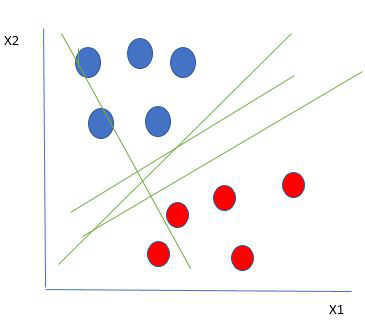Linearly Separable Data points

From the figure above its very clear that there are multiple lines (our hyperplane here is a line because we are considering only two input features x1, x2) that segregates our data points or does a classification between red and blue circles. So how do we choose the best line or in general the best hyperplane that segregates our data points.

Selecting the best hyper-plane:

One reasonable choice as the best hyperplane is the one that represents the largest separation or margin between the two classes.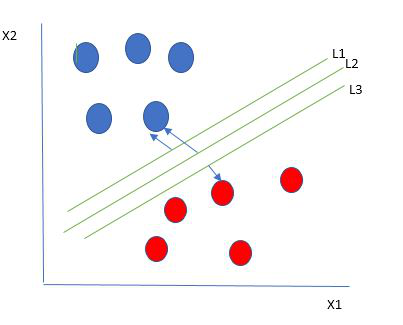So we choose the hyperplane whose distance from it to the nearest data point on each side is maximized. If such a hyperplane exists it is known as the maximum-margin hyperplane/hard margin. So from the above figure, we choose L2.

Let’s consider a scenario like shown below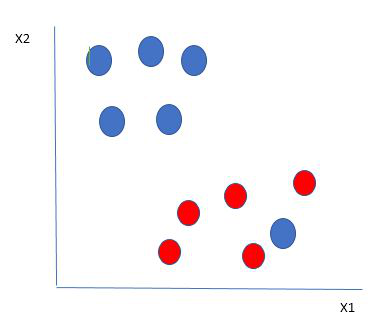Here we have one blue ball in the boundary of the red ball. So how does SVM classify the data? It’s simple! The blue ball in the boundary of red ones is an outlier of blue balls. The SVM algorithm has the characteristics to ignore the outlier and finds the best hyperplane that maximizes the margin. SVM is robust to outliers.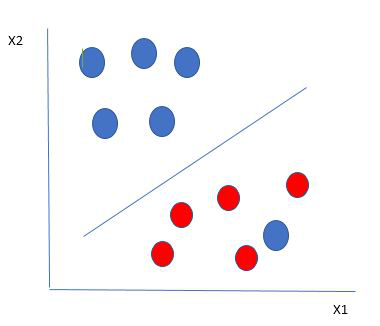So in this type of data points what SVM does is, it finds maximum margin as done with previous data sets along with that it adds a penalty each time a point crosses the margin. So the margins in these type of cases are called soft margin. When there is a soft margin to the data set, the SVM tries to minimize (1/margin+∧(∑penalty)). Hinge loss is a commonly used penalty. If no violations no hinge loss.If violations hinge loss proportional to the distance of violation.

Till now, we were talking about linearly separable data(the group of blue balls and red balls are separable by a straight line/linear line). What to do if data are not linearly separable?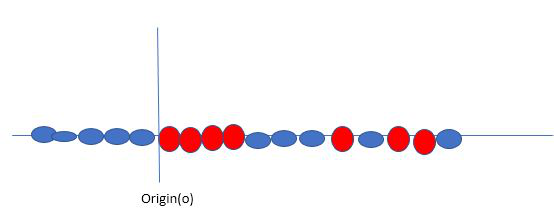Say, our data is like shown in the figure above.SVM solves this by creating a new variable using a kernel. We call a point xi on the line and we create a new variable yi as a function of distance from origin o.so if we plot this we get something like as shown below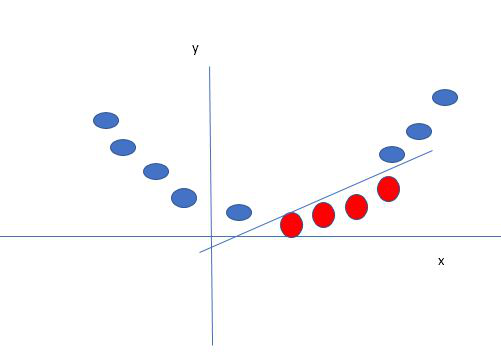In this case, the new variable y is created as a function of distance from the origin. A non-linear function that creates a new variable is referred to as kernel.

SVM Kernel:

The SVM kernel is a function that takes low dimensional input space and transforms it into higher-dimensional space, ie it converts not separable problem to separable problem. It is mostly useful in non-linear separation problems. Simply put the kernel, it does some extremely complex data transformations then finds out the process to separate the data based on the labels or outputs defined.

• Effective in high dimensional cases
• Its memory efficient as it uses a subset of training points in the decision function called support vectors
• Different kernel functions can be specified for the decision functions and its possible to specify custom kernels

SVM implementation in python:

Objective: Predict if cancer is beningn or malignant.

Using historical data about patients diagnosed with cancer, enable the doctors to differentiate malignant cases and benign given the independent attributes.

## Python

 `# import libraries``import` `pandas as pd``import` `numpy as np``import` `seaborn as sns``import` `matplotlib.pyplot as plt``%``matplotlib inline``# Importing Data file``data ``=` `pd.read_csv(``'bc2.csv'``)``dataset ``=` `pd.DataFrame(data)``dataset.columns`

Output:

```Index(['ID', 'ClumpThickness', 'Cell Size', 'Cell Shape', 'Marginal Adhesion',
'Single Epithelial Cell Size', 'Bare Nuclei', 'Normal Nucleoli', 'Bland Chromatin',
'Mitoses', 'Class'], dtype='object')```

## Python

 `dataset.info()`

Output:

```<class 'pandas.core.frame.DataFrame'>
RangeIndex: 699 entries, 0 to 698
Data columns (total 11 columns):
ID                             699 non-null int64
ClumpThickness       699 non-null int64
Cell Size                    699 non-null int64
Cell Shape                 699 non-null int64
Single Epithelial Cell Size    699 non-null int64
Bare Nuclei                    699 non-null object
Normal Nucleoli            699 non-null int64
Bland Chromatin           699 non-null int64
Mitoses                         699 non-null int64
Class                             699 non-null int64
dtypes: int64(10), object(1)
memory usage: 60.1+ KB```

## Python

 `dataset.describe().transpose()`

Output:

## Python

 `dataset ``=` `dataset.replace(``'?'``, np.nan)``dataset ``=` `dataset.``apply``(``lambda` `x: x.fillna(x.median()),axis``=``0``)`` ` `# converting the hp column from object 'Bare Nuclei'/ string type to float``dataset[``'Bare Nuclei'``] ``=` `dataset[``'Bare Nuclei'``].astype(``'float64'``)  ``dataset.isnull().``sum``()`

Output:

```ID                                               0
ClumpThickness                         0
Cell Size                                      0
Cell Shape                                   0
Single Epithelial Cell Size            0
Bare Nuclei                                  0
Normal Nucleoli                          0
Bland Chromatin                         0
Mitoses                                        0
Class                                             0
dtype: int64```

## Python

 `from` `sklearn.model_selection ``import` `train_test_split`` ` `# To calculate the accuracy score of the model``from` `sklearn.metrics ``import` `accuracy_score, confusion_matrix`` ` `target ``=` `dataset[``"Class"``]``features ``=` `dataset.drop([``"ID"``,``"Class"``], axis``=``1``)``X_train, X_test, y_train, y_test ``=` `train_test_split(features,target, test_size ``=` `0.2``, random_state ``=` `10``)``from` `sklearn.svm ``import` `SVC`` ` `# Building a Support Vector Machine on train data``svc_model ``=` `SVC(C``=` `.``1``, kernel``=``'linear'``, gamma``=` `1``)``svc_model.fit(X_train, y_train)`` ` `prediction ``=` `svc_model .predict(X_test)``# check the accuracy on the training set``print``(svc_model.score(X_train, y_train))``print``(svc_model.score(X_test, y_test))`

Output:

```0.9749552772808586
0.9642857142857143```

## Python

 `print``(``"Confusion Matrix:\n"``,confusion_matrix(prediction,y_test))`

Output:

```Confusion Matrix:
[[95  2]
[ 3 40]]```

## Python

 `# Building a Support Vector Machine on train data``svc_model ``=` `SVC(kernel``=``'rbf'``)``svc_model.fit(X_train, y_train)`

Output:

```SVC(C=1.0, cache_size=200, class_weight=None, coef0=0.0,
decision_function_shape='ovr', degree=3, gamma='auto_deprecated',
kernel='rbf', max_iter=-1, probability=False, random_state=None,
shrinking=True, tol=0.001, verbose=False)```

## Python

 `print``(svc_model.score(X_train, y_train))``print``(svc_model.score(X_test, y_test))`

Output:

```0.998211091234347
0.9571428571428572```

## Python

 `#Building a Support Vector Machine on train data(changing the kernel)``svc_model  ``=` `SVC(kernel``=``'poly'``)``svc_model.fit(X_train, y_train)`` ` `prediction ``=` `svc_model.predict(X_test)`` ` `print``(svc_model.score(X_train, y_train))``print``(svc_model.score(X_test, y_test))`

Output:

```1.0
0.9357142857142857```

## Python

 `svc_model ``=` `SVC(kernel``=``'sigmoid'``)``svc_model.fit(X_train, y_train)`` ` `prediction ``=` `svc_model.predict(X_test)`` ` `print``(svc_model.score(X_train, y_train))``print``(svc_model.score(X_test, y_test))`

Output:

```0.3434704830053667
0.32857142857142857```

My Personal Notes arrow_drop_up